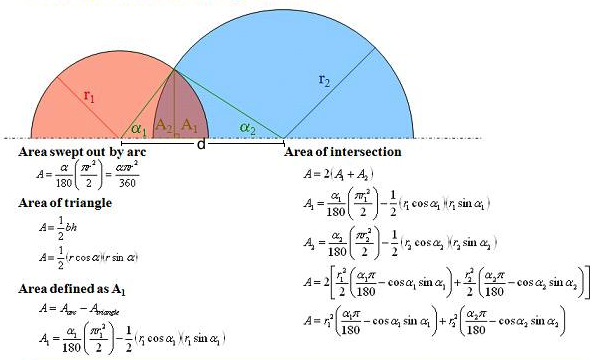Engineering ToolBox - Resources, Tools and Basic Information for Engineering and Design of Technical Applications!

# Area of Intersecting Circles

## Calculate area of intersecting circles

The area of intersecting circles can be calculated asThanks to Eric Eberly for providing this information.

If radii r1, r2 and distance d between the centers are given - then the angles α1 and α2 can be calculated as

α1 = acos[(d2 + r12 - r22)/(2 d r1)]

α2 = acos[(d2 + r22 - r12)/(2 d r2)]

## Related Topics

• ### Mathematics

Mathematical rules and laws - numbers, areas, volumes, exponents, trigonometric functions and more.

## Related Documents

• ### Circle - Equation

The equation for a circle
• ### Circle - the Chord Lengths when Divided in to Equal Segments

Calculate chord lengths when dividing the circumference of a circle into an equal number of segments.
• ### Circles Outside a Circle

Calculate the numbers of circles on the outside of an inner circle - like the geometry of rollers on a shaft.
• ### Circles within a Rectangle - Calculator

Calculate the maximum number of circles within a rectangle - can be used to calculate the numbers of pipes or wires in a conduit or similar.
• ### Elementary Curves

Ellipse, circle, hyperbola, parabola, parallel, intersecting and coincident lines.
• ### Elementary Surfaces

Ellipsoid, sphere, hyperboloid, cone and more.
• ### Equal Areas - Circles vs. Squares

Radius and side lengths of equal areas, circles and squares.
• ### Geometric Shapes - Areas

Areas, diagonals and more - of geometric figures like rectangles, triangles, trapezoids ..
• ### Smaller Rectangles within a Larger Rectangle

The maximum number of smaller rectangles - or squares - within a larger rectangle (or square).

## Engineering ToolBox - SketchUp Extension - Online 3D modeling!

Add standard and customized parametric components - like flange beams, lumbers, piping, stairs and more - to your Sketchup model with the Engineering ToolBox - SketchUp Extension - enabled for use with older versions of the amazing SketchUp Make and the newer "up to date" SketchUp Pro . Add the Engineering ToolBox extension to your SketchUp Make/Pro from the Extension Warehouse !

We don't collect information from our users. More about

## Citation

• The Engineering ToolBox (2009). Area of Intersecting Circles. [online] Available at: https://www.engineeringtoolbox.com/area-intersecting-circles-d_1571.html [Accessed Day Month Year].

Modify the access date according your visit.

9.19.12